# Ⅲ. Single-currency margin: cross margin tradingOKX

Single-currency margin: In this mode, users can trade across all business lines simultaneously, including spot, margin, futures, perpetual swap, and options. Users only need to transfer assets into the cross margin account. In single-currency cross margin, all trading products settled with the same currency can share the same total margin, and the generated profits and losses can be offset.

In single-currency cross margin, the risks of the positions settled with the same currency will be measured in whole. When the equity of a certain currency is insufficient, it may result in partial liquidation, full liquidation, or equity loss of all the positions settled with this currency. If users want to segregate the risk of each position, isolated margin mode will be a good choice.

Assets

In single-currency margin, the assets will be displayed as below: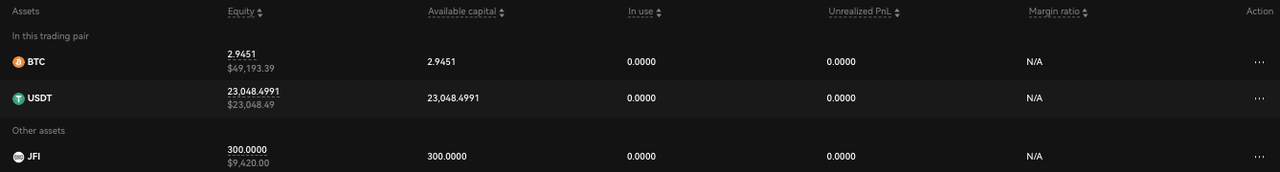Term Explained
Equity Your individual asset balance plus unrealized profit and loss from all positions in single-currency margin mode.

Equity = Balance in the single-currency margin account + PnL in cross margin positions + Margin balance in isolated positions + PnL in isolated positions + Options market value
Available capital The amount of assets in the single-currency account and can be used for margin, futures, perpetual swap, and options (short positions) trading with the cross margin mode.

Available capital = Max (0, balance in cross positions + unrealized P&L in cross margin positions - In use amount)
Available balance The amount of asset that can be used for isolated positions, spot and options (long positions) trading in single-currency account.

Note: the above is only for order calculation and will not displayed on the website.
In use The amount of certain crypto used as cross margin in pending orders and positions, isolated margin in pending orders, and used in trading bots
Unrealized P&L The sum of the Unrealized P&L of all margin and derivatives positions including cross positions and isolated positions that share the same settlement currency.

Unrealized P&L = Unrealized P&L of cross margin positions + Unrealized P&L of isolated margin positions.

1. Unrealized P&L of cross positions = Unrealized P&L of cross margin positions + Unrealized P&L of futures positions in cross margin mode+ Unrealized P&L of perpetual positions in cross margin mode + Unrealized P&L of options positions in cross margin mode

2. Unrealized P&L of isolated positions = Unrealized P&L of margin positions in isolated margin mode + Unrealized P&L of futures positions in isolated margin mode + Unrealized P&L of perpetual positions in isolated margin mode + Unrealized P&L of options positions in isolated margin mode
Leverage The leverage of a single currency, leverage = position value/ (balance in cross positions + unrealized P&L in cross margin positions).

Position value: the sum of position value of all positions that are settled in this currency

Calculation of position value

1. Coin-margined Futures and swaps:
Position value = face value * |number of contracts| * multiplier / mark price
2. USDT-margined Futures and swaps:
Position value = face value * |number of contracts| * multiplier * mark price
3. Margin
(1) Long positions with trading currency as margin currency, and liability calculated in quote currency.
Position value = |liability + interest|/ mark price
(2) Short positions with trading currency as margin currency, and liability calculated in trading currency.
Position value = |liability + interest|
(3) Short positions with quote currency as margin currency, and liability calculated in trading currency.
Position value = |liability + interest| * mark price
(4) Long positions with quote currency as margin currency, and liability calculated in quote currency.
Position value = |liability + interest|
4. Options
Position value = face value * |number of contracts| * multiplier
Margin Level The index for measuring the risk of a certain asset in the account.

Margin level= (balance of a certain asset in single -currency cross margin account + P&L in cross margin positions - the amount of sold asset in pending orders - the amount required for options buy orders - the amount required for pending orders in isolated margin mode – order fees)/(Maintenance margin + liquidation fees)

The maintenance margin is the sum of the maintenance margin of futures, margin, perpetual, and options positions. Pending orders are also included.

The liquidation fees are also the sum of the fees charged for margin, futures, perpetual and options transactions. Pending orders are also included.

These settings are to avoid liquidations caused by a sudden change in the account risk level after the pending order is filled.
Total equity The net value of all crypto assets in the user account that converted into fiats.

Total equity = Sum (currency equity * currency price)

The currency price is based on the USD index price of the currency on OKX. If a currency does not have a USD index price, then apply: the spot price of the pair (currency/USDT) on OKX * USDT price in USD

In single-currency margin account, users can choose to trade in cross margin mode or isolated margin mode. In cross margin mode, all trading products settled with the same currency can share the same total margin, and the generated profits and losses can be offset. In isolated margin mode, the risk of each position, as well as the profit and loss, are separated.

1. Trading rules for single-currency cross margin mode

1. When users conduct futures, perpetual, options(short positions), and margin transactions in single-currency cross margin mode, the available equity of the currency in the account should be greater than or equal to the amount required for the order.

2. When users conduct spot or options(long positions) transactions in single-currency cross margin mode, the available balance of the currency in the account should be greater than or equal to the amount required for the order.

Note: Available balance refers to the amount of assets that can be used to open isolated positions and perform spot and options (long positions) trading with the single-currency account.

If a user's BTC holdings in single-currency cross margin positions and pending orders are as below: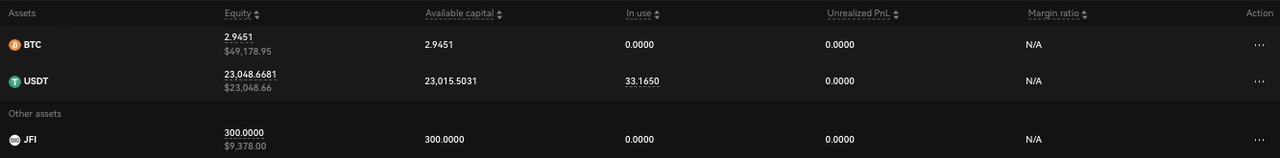Margin (Isolated) Margin (Cross) Futures (Cross)
Underlying BTCUSDT Margin Underlying BTCUSDT Margin Underlying BTCUSD Quarterly
Leverage 5 Long Leverage 5 Long Leverage 1 Long
Position margin 100 BTC Position margin 100 BTC Position margin 10 BTC
Pending order margin 200 BTC Pending order margin 200 BTC Pending order margin 20 BTC
UPL 10 UPL 10 UPL 5 BTC
MRR 1% MRR 1% MRR 1%

And if the user conducts the margin trading in single-currency cross margin mode with the holdings as above, the margin verification for placing an order will be as follows:

Then when the user opens a long position with 200 BTC and 5x leverage, the amount of required margin for this order will be 200 / 5 = 40 BTC;

On-hold equity = margin required for futures positions in cross margin mode + pending order margin for futures in cross margin mode + margin required for margin positions in cross margin mode + pending order margin for margin trading in cross margin mode + pending order margin required for isolated margin mode = 10 + 20 + 100 + 200 + 200 = 530 BTC;

Available equity = Max (0, balance in cross margin account + Unrealized P&L in cross margin positions - In use) = max(0, 700 + 10 + 5 - 530 ) = 185 BTC;

If the BTC available equity > the amount of BTC required for the order, it means the order is placed successfully.

If the user conducts the futures trading in single-currency cross margin mode with the holdings as above, the margin verification for placing an order will be as follows:

If the user longs 100,000 weekly futures contracts with 5x leverage at a price of 10,000 USDT/BTC, the required margin amount = face value * number of contracts * contract multiplier / order price / leverage multiplier = 100000 * 100 * 1 / 10000 / 5 = 200 BTC;

On - hold equity = margin required for futures positions in cross margin mode + pending order margin for futures in cross margin mode + margin required for margin positions in cross margin mode + pending order margin for margin trading in cross margin mode + pending order margin required for isolated margin mode = 10 + 20 +100 +200 + 200 = 530 BTC;

Available equity = Max (0, balance in cross margin account + Unrealized P&L in cross margin positions - In use) = max(0, 700 + 10 + 5 - 530 ) = 185 BTC;

That is, the BTC available margin is less than the amount required for the order, so the order fails.

Trading rules for single-currency cross margin mode

1. Margin positions in cross margin mode

2. If a user trades a certain margin pair in the single-currency cross margin mode, the margin positions will be displayed as below: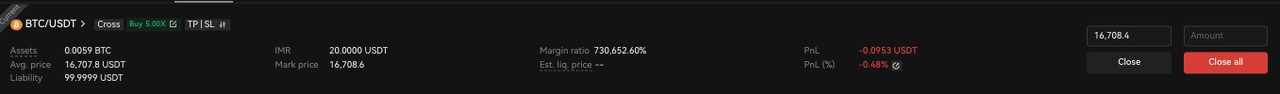Term Explained
Assets The amount of positive assets in the position (excluding margin).
Available asset The amount of positive assets to close a position. For detailed info, please refer to the following.
Liability Initial liabilities + deducted interest
- Long position: Liability is calculated in the quote currency.
- Short position: Liability is calculated in the trading currency.
Interest Generated interest that has not been deducted yet.
Avg.open price (Original holding amount * Original Avg.open price + new holding amount * filled price) / (Original holding amount + new holding amount)
Est.liquidation price The underlying price when the cross position of a single- currency account meets the liquidation conditions.
The price is indicative.
Note: When there is an options position or a margin position of non-USDT pair in single-currency cross margin mode, the est. liquidation price can't be calculated.
In the USDT single-currency cross margin mode, if there are contracts or margin positions with different underlyings, the est. liquidation price cannot be calculated either.
P&L Unrealized profit or loss of the current position.Calculation formula of P&L:
1. Long positions with the trading currency serving as the margin currency, and the P&L is calculated in the trading currency.P&L = Total assets - (liability + interest) / mark price
2. Long positions with the quote currency serving as the margin currency, and the P&L is calculated in the quote currency.P&L = Total assets * mark price - (liability + interest)
3. Short positions with the quote currency serving as the margin currency, and the P&L is calculated in the quote currency.P&L = total assets - (liability + interest) * mark price
4. Short positions with the trading currency serving as the margin currency, and the P&L is calculated in the trading currency.
P&L = Total assets / marked price - (liability + interest)
P&L ratio P&L/initial margin
Initial margin 1. Long positions with trading currency as margin currency, and liability calculated in quote currency.Initial margin = (liability + interest) / (mark price * leverage)
2. Short positions with trading currency as margin currency, and liability calculated in trading currency.Initial margin = (liability + interest) / leverage
3. Short positions with quote currency as margin currency, and liability calculated in trading currency.Initial margin = (liability + interest) * mark price / leverage
4. Long positions with quote currency as margin currency, and liability calculated in quote currency.Initial margin = (liability + interest) / leverage
Maintenance margin 1. Long positions with trading currency as margin currency.Maintenance margin = (liability + interest) * maintenance margin ratio / mark price
2. Long positions with quote currency as margin currency.Maintenance margin = (liability + interest) * maintenance margin ratio
3. Short positions with quote currency as margin currency.Maintenance margin = (liability + interest) * maintenance margin ratio * mark price
4. Short positions with trading currency as margin currency.
Maintenance margin = (liability + interest) * maintenance margin ratio

2.1.2 Principle of initial margin: both tokens of a pair can be used as margin for long/short positions.

Examples:

1. As to BTC/USDT pair, you can choose either BTC or USDT as margin currency to open long/short margin positions in single-currency cross mode.

2. Now open a long position of 1BTC under 10X leverage. If the user chooses to take BTC as the margin currency, a margin of 0.1 BTC is required (the available equity in single-currency account should be greater than or equal to 0.1 BTC), the filled price is 10,000 USDT/BTC, and 10,000 USDT needs to be borrowed; no currency will be borrowed if the transaction is not filled. No interest is accrued, but the margin will be on-hold.

3. After the transaction is filled, a long position will be opened: the total asset of this position is 1BTC, while its liability is 10,000USDT. The margin of 0.1BTC is in the account balance, and will not be transferred to single-currency margin position asset (It is different from isolated position, where margin for isolated position will be transferred to the position asset).

3. Closing positions

3.1 Position assets and margin currency are the same---use the trading currency as margin to open long, or use the quote currency as margin to open short.

1. Principle of closing positions: Only the available asset can be used for closing a position. Users can close the position when they pay off the liability; Users can also choose whether to use "reduce only" when closing a position.

2. The available asset formula for closing positions:

The main difference from the isolated margin: the available asset can be used for closing the position is the total asset of the isolated margin positions, while in single-currency cross margin mode (where position assets and margin currencies are the same), the available asset that can be used for closing the position may be the sum of total position asset and part of the equity in account balance.

Fundamentally, closing a margin position cannot affect the risks of other positions in a single-currency cross margin mode. When the total equity is greater than or equal to the initial margin required for the account, the available asset for closing a margin position can include the initial margin of this position; when the equity is less than the maintenance margin required for the account, the available asset for closing a margin position can include the maintenance margin of this position.

a) Closing long positions with liability calculated in quote currency:

• Account equity ≥ account initial margin, the available asset for closing margin positions = (|liability + interest| of this margin position) * (1 + IMR% of this margin position) / mark price

• Account equity < account maintenance margin, the available asset for closing margin positions = (|liability + interest| of this margin position) * (1 + MMR% of this margin position) / mark price

b) Closing short positions with liability calculated in trading currency:

• Account equity ≥ account initial margin, the available asset for closing margin positions = (|liability + interest| of this margin position) * (1 + IMR% of this margin position) * mark price

• Account equity < account maintenance margin, the available asset for closing margin positions = (|liability + interest| of this position) * (1 + MMR% of this position) * mark price

1. The rules of closing positions are the same as those of isolated positions, except the available asset formula.
No. Mode Closing method Rule Example
1 Close in Position Market close all 1. Only pay off the liabilities, and the remaining assets will be transferred to the single-currency account balance.
2. Only the available assets can be used to close the positions.
3. The default setting is “reduce only".
The current margin position is long position—Its available asset is 2 BTC, liability 10,000 USDT, and interest 10 USDT.
1. The system will calculate how much USDT needs to be bought to close the position and pay off liabilities (Interest and fees will also be included). If it is 10,020 USDT, the system will sell 2 BTC at the market price, and then stop it when you receive 10,020 USDT. Due to transaction accuracy, it may exceed a little.
2. Assuming the average filled price is 10,000 USDT, then buying 10,020 USDT requires 1.002 BTC, and the remaining 0.998 BTC will not be sold.
3. After closing the position, the remaining 0.998 BTC will be transferred to the BTC single-currency account balance, and due to accuracy reasons, the remaining USDT will also be transferred to the USDT single-currency account balance after the liability is paid off.
Closing at limit price 1. You can buy assets that exceed the liabilities. Once the liability is paid off, the margin position will be closed. The oversold assets and remaining ones will be transferred to the single-currency account balance.
2.Only the available asset can be used to close the position.
3.The default setting is "reduce only".
The current margin position is long position—Its available asset is 2 BTC, liability 10,000 USDT, and interest 10 USDT
1. Sell 0.5 BTC at the filled price of 10,000 USDT, then 5,000 USDT is bought. After deducting the fee of 5 USDT and interest of 10 USDT, the remaining 4,985 USDT will be used to pay off the liability. With a remaining liability of 5,015 USDT, the position still exists.
2. After partially closing the position: the position asset is 1.5 BTC, liability 5,015 USDT, and interest 0 USDT.
3. Sell 1 BTC at the filled price of 10,000 USDT, then 10,000 USDT is bought. After deducting the fee of 15 USDT, the remaining 9,985 USDT will be used to pay off the liability of 5,015 USDT. Since liability has been paid off, the position disappeared;
4. The remaining assets of 0.5 BTC and 4,970 USDT will be transferred to the account balance of BTC and USDT single-currency account separately.
2 Close in close short/close long area Reduce only The same as those of [Close in Position] -
Reduce + reverse position 1. When the liability is paid off, the margin position will disappear. The oversold asset will be used to open a reverse position;
2. Only the available asset can be used to close the position, and the margin for opening a reverse position is the available equity of the corresponding single-currency account.
The current margin position is a short position—Its available asset is 30,000 USDT, liability 2 BTC, with interest and transaction fees temporarily ignored.
1. Buy 1 BTC at the filled price of 10,000 USDT. This order uses assets of 10,000 USDT to pay off the liability of 1 BTC;
2. After partially closing the position: the remaining asset of it is 20,000 USDT, and the remaining liability is1 BTC. Since the liability has not been paid off, the position still exists;
3. Buy 1.5 BTC at the filled price of 10,000 USDT. 1.5 BTC > liability of 1 BTC. After the order is filled, the position will be closed first, and then a reverse position will be opened.
4. The asset of 10,000 USDT is used to paid off the liability of 1 BTC, and after the long position is closed, the remaining 10,000 USDT asset on the position will be transferred to the USDT single-currency account balance;
5. The reverse position of 0.5 BTC will use 1,000 USDT in the single-currency account balance as its margin, and 5,000 USDT will be borrowed to open a reverse long position;
6. At this time, the position asset of this margin long position is 0.5 BTC, and liability is 5,000 USDT.

3.2 Position assets and margin currencies are different---use the trading currency as margin currency to open short, or use the quote currency as margin currency to open long.

1. Principle of closing positions: only position assets can be used to close a position. If the position assets are sold out, the position will be closed. If the liability is not paid off, the equity in the account will be used to directly pay off the remaining liabilities; Users can choose whether to use "reduce only".

2. The available asset that can be used to close the position = position assets.

No. Mode Closing method Rule Example
1 Close in Position Market close all 1. The position will be closed when all position assets are sold at market price.
2. The default setting is “reduce only”.
The current margin position is a long position—Its position asset is 2 BTC, liability 10,000 USDT, with interest and fees temporarily ignored.Its margin currency is USDT, and its margin is in USDT single-currency account balance.
1. The system will sell 2 BTC. If the average filled price is 9,000 USDT, a total of 18,000 USDT will be bought. The liability of 10,000 USDT will be paid off. The remaining 8,000 USDT will be transferred to USDT account balance and the position will be closed.
2. If the average filled price is 2,000 USDT, a total of 4,000 USDT will be bought. The remaining liability will be 6,000 USDT, which will be paid off by USDT account balance. Then the position will be closed.
Closing at limit price 1.The position will be closed when all position assets are sold at limit price.
2. The default setting is “reduce only”
The current margin position is a long position—Its position asset is 2 BTC, liability 10,000 USDT, with interest and fees temporarily ignored.Its margin currency is USDT, and its margin is in USDT single-currency account balance.
1. Sell 1BTC at the average filled price of 15,000 USDT, a total of 15,000 USDT will be bought. The liability of 10,000 USDT will be paid off. The remaining 5,000 USDT will be transferred to the USDT account balance. The position is not closed yet because there is still 1 BTC position asset, which is different from the margin currency.
2. Sell the remaining 1 BTC position asset at the filled price of 10,000 USDT, then a total of 10,000 USDT will be bought. Since liability has been paid off, 10,000 USDT will be transferred to the USDT account balance. Because the position asset is 0, the position is closed.
2 Close in close short/close long area Reduce only The same as those of [Close in Position] -
Reduce only + reverse position 1. The position will be closed when all position assets are sold. The remaining assets will be used to open a reverse position;
2. The available asset is position assets, and the margin for opening a reverse position is the available equity of the corresponding currency of the account.
a position asset of 5,000 USDT. After the order is filled, the position will be closed first, and then a reverse position will be opened; 0.5 BTC will be bought by a position asset of 5,000 USDT, and transferred to BTC single-currency account balance. 10,000 USDT will be borrowed to buy 1 BTC to open a reverse long position, and 0.1 BTC in the single-currency account balance will be used as margin. At this time, the position asset of this margin long position is 1 BTC, and liability of it is 10,000 USDT. The current margin position is a short position—Its position asset is 30,000 USDT, liability 2 BTC, with interest and fees temporarily ignored.
1. Buy 2.5 BTC at the filled price of 10,000 USDT. This order uses position asset of 25,000 USDT to pay off the liability of 2 BTC. The remaining 0.5 BTC will be transferred to BTC single-currency account balance. Although the liability is paid off, the position is not closed yet with a position asset of 5,000 USDT.
2. Buy 1.5 BTC at the filled price of 10,000 USDT. 15,000 USDT> a position asset of 5,000 USDT. After the order is filled, the position will be closed first, and then a reverse position will be opened;
3. 0.5 BTC will be bought by a position asset of 5,000 USDT, and transferred to BTC single-currency account balance.
4. 10,000 USDT will be borrowed to buy 1 BTC to open a reverse long position, and 0.1 BTC in the single-currency account balance will be used as margin.
5. At this time, the position asset of this margin long position is 1 BTC, and liability of it is 10,000 USDT.

2.2 Perpetual/futures in cross margin mode

In single-currency margin mode, perpetual/futures support both Hedge mode and One-way mode, as shown in the following figures:

(1) Hedge mode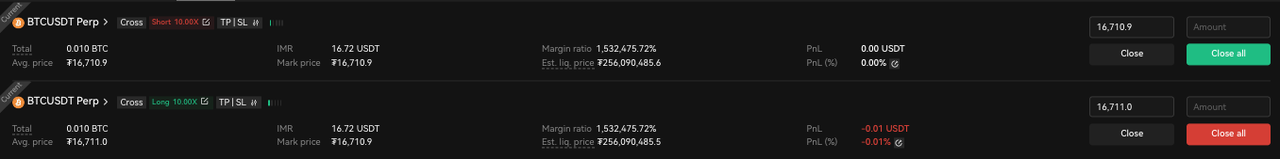(2) One-way mode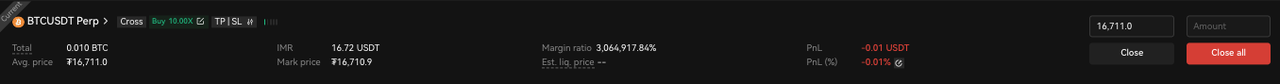Term Definition
Total For the One-way mode, the total of long positions is a positive number, and the total of short positions is a negative number.
Avail. Only shown in Hedge mode Avail. = total positions – positions of pending close orders
P&L Unrealized profit or loss of current position
1. Coin-margined futures/perpetual swap
P&L of long positions = face value * |number of contracts| * multiplier * (1 / avg. open price – 1 / mark price)
P&L of short positions = face value * |number of contracts| * multiplier * (1 / mark price – 1 / avg. open price)
2. USDT-margined futures/perpetual swap
P&L of long positions = face value * |number of contracts| * multiplier * (mark price – avg. open price) P&L of short positions = face value * |number of contracts| * multiplier * (avg. open price - mark price)
P&L ratio P&L / initial margin
Initial margin 1. Coin-margined futures/perpetual swap Initial margin = face value * |number of contracts| * multiplier / (mark price * leverage)
2. USDT-margined futures/perpetual swap Initial margin = face value * |number of contracts| * multiplier * mark price / leverage
Maintenance margin 1. Coin-margined futures/perpetual swap
Maintenance margin = face value * |number of contracts| * multiplier * maintenance margin ratio / mark price
2.USDT-margined futures/perpetual swap
Maintenance margin = face value * |number of contracts| * multiplier * maintenance margin ratio * mark price

2.3 Options in cross margin mode

In single-currency margin mode, you will be able to open long and short positions of options: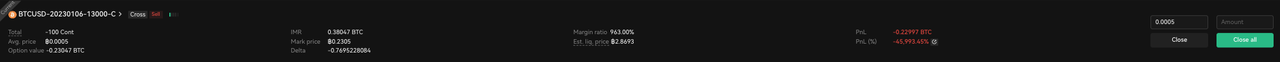Term Definition
Total The total of long positions is a positive number, and the total of short positions is a negative number.
Options value If the unit for calculating the price is currency, then options value = total positions * mark priceIf the unit for calculating the price is the number of contracts, then options value = total positions * mark price * multiplier
P&L Unrealized profit or loss of current positionP&L = (mark price - avg. open price) * total positions * multiplier
P&L ratio P&L of long positions = (mark price – avg. open price) / avg. open price P&L of short positions = (avg. open price - mark price) / avg. open price
Initial margin The initial margin for long positions is 0. As to initial margin of short positions, please refer to Introduction to the calculation of options margin.
Maintenance margin The maintenance margin for long positions is 0. As to maintenance margin of short positions, please refer to Introduction to the calculation of options margin.

Risk assessment

The single-currency margin mode applies a double-layer risk assessment measure. The first layer is "order cancellation by risk control system", and the other is "pre-liquidation verification". This measure ensures a smooth trading experience, prevents the pending orders from being completely canceled, and prevents the positions from being partially or even fully liquidated due to insufficient margin.

Order cancellation by risk control system

If a user's account is assessed as risky but not risky enough to trigger "pre-liquidation verification", the" order cancellation by risk control system" will be executed, and some pending orders will be canceled to ensure the account stays within a safe risk control level, which can prevent the pending orders from being canceled because of a sudden triggering of "pre-liquidation verification".

Rules for "order cancellation by risk control system" in single-currency margin mode

1. When (Available equity of the currency in single-currency account - used balance) < the required maintenance margin for all the positions + the initial margin for the pending orders of opening positions in cross margin mode + the pending orders fees, all the pending orders that will increase the used equity amount will be cancelled.

(Including the opening orders of futures, perpetual, options, and margin in both cross margin and isolated margin mode, as well as the pending orders for selling the currency).

1. When the available balance <0, all open orders inisolated margin mode, pending orders for selling the currency, and options buy orders will be canceled.

Pre-liquidation verification

The liquidation that happens in single-currency margin mode is triggered depending on whether or not the margin level reaches 100%. In the single-currency cross margin mode, the margin level is an indicator calculated from the cross margin account equity and maintenance margin in a certain currency. Generally, the higher the account equity, and the lower the maintenance margin, the lower the risk.

When the margin level of a certain currency in single-currency cross margin mode is < 300%, the system will send a liquidation alert to the account, warning users of the risk of liquidation. 300% is set as the pre-alert parameter, and OKX reserves the right to adjust this parameter according to the actual situation.

When the margin level of a certain currency in single-currency cross margin mode is <= 100%, the system will cancel the orders according to the following rules, that is, order cancellation by pre-liquidation system:

Business line Mode Single-currency cross margin
Futures&perpetual Hedge mode Cancel all unfilled pending orders (including algo orders) in cross margin mode, the limit orders and stop-loss orders of opening positions in isolated margin mode; the stop-loss orders of closing positions in isolated margin mode and algo orders except stop orders in isolated margin mode will not be canceled.
One-way mode Cancel all unfilled pending orders (including algo orders) in cross margin mode, and the limit orders of opening positions in isolated margin mode; algo orders in isolated margin mode will not be canceled.
Margin - Cancel all unfilled pending orders (including algo orders) in cross margin mode, and the limit orders with the same direction of current positions in isolated margin mode; algo orders in isolated margin mode will not be canceled.
Options - Cancel all unfilled pending orders in cross margin mode and orders of opening positions in isolated margin mode.

If the margin level is still <= 100% after the orders are canceled, the account will trigger a liquidation.

The partial liquidation process can be divided into three phases. In each stage, the positions to be partially liquidated will be handed over to the liquidation engine at the mark price, and a certain amount of maintenance margin will be charged (It is determined by your position tier, which will make up for the loss of the liquidation engine, and the remaining will be injected into the insurance fund, which will not get additional liquidation fee). The option long position will not be partially liquidated.

Three phases of partial liquidation:

1）First, partial liquidation will start with the long and short reverse positions of the same contract under the Hedge mode.

1. When there is no position described in phase 1), or all positions described in phase 1) are partially liquidated, and the account is not restored to a safe state, the system will try to keep the total delta value of the account unchanged while attempting to reduce the overall risk of the account. That is, partially liquidate the long and short hedged positions in terms of delta value (Delta refers to a rate of change in the position value of a contract per change in the index price. When the two changes are in the same direction, the delta is positive. Otherwise, it is negative. The greater the change in the position value of a contract per change in the index price, the greater the absolute value of delta). If multiple positions meet the delta long and short offsetting conditions, the system will first partially liquidate the position with a larger maintenance margin.

2. When there is no position described in 2), or all positions described in 2) are partially liquidated (that is, the total delta value of the account cannot be kept almost unchanged at this time, while the account risk cannot be reduced), and the account has not been restored to safety status, the system will try to partially liquidate the remaining unhedged positions, and will give priority to those with the best risk reduction effect. Each time you partially liquidate your position, the position tier will be reduced by one level until the account is safe again.

For example, the current price of futures contract BTC-USD-0925 is 50000 USD/BTC, assuming the account assets are as follows:

Balance 5 BTC Single-currency cross margin
Positions Options: BTCUSD-20200925-65000-C -100 conts, Position delta <0
Futures: BTCUSD0925 +1000 conts, Position delta > 0
Options: BTCUSD-20200925-55000-P -500 conts, Position delta >0
Cancel all unfilled pending orders (including algo orders) in cross margin mode, the limit orders and stop-loss orders of opening positions in isolated margin mode; the stop-loss orders of closing positions in isolated margin mode and algo orders except stop orders in isolated margin mode will not be canceled.
Futures: BTCUSD1225 +100 conts, Order price at 9,000
Perpetual swap: BTCUSD perpetual swap -100 conts, Order price at 8,000
Cancel all unfilled pending orders (including algo orders) in cross margin mode, and the limit orders of opening positions in isolated margin mode; algo orders in isolated margin mode will not be canceled.
Margin - Cancel all unfilled pending orders (including algo orders) in cross margin mode, and the limit orders with the same direction of current positions in isolated margin mode; algo orders in isolated margin mode will not be canceled.
Options - Cancel all unfilled pending orders in cross margin mode and orders of opening positions in isolated margin mode.

The liquidation condition is triggered when the margin level is 93%. After all the pending orders are canceled, the margin level of the position turns 95%. Then the position goes into liquidation status. At this moment, the available equity in the cross margin account is 9.5 BTC, and the maintenance margin is 10 BTC.

The position does not contain the long and short reverse positions of the same contract in the long and short mode, but contains the long and short hedged positions in terms of delta value.

After calculation, the futures BTCUSD0925 will be liquidated by 500 conts of long positions, and the options BTCUSD-20200925-65000-C will be liquidated by 100 conts of short positions. After liquidation, the account equity is 9.4 BTC, and the maintenance margin is 9.6 BTC.

If the account is not restored to a safe state and there is no hedged position in the single currency account (all short positions of BTCUSD-20200925-65000-C are liquidated, and the delta value of all remaining positions is positive), please apply liquidation logic to unhedged positions.